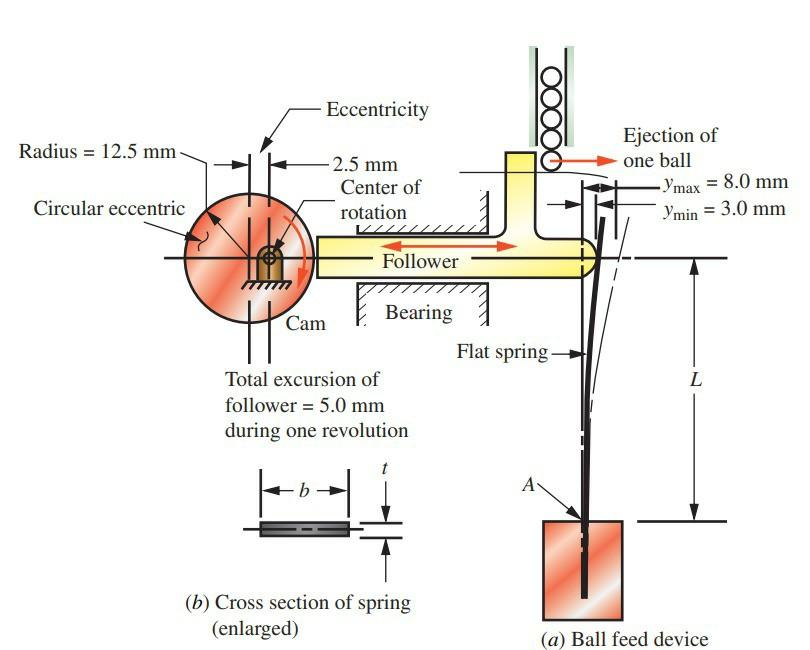# For the flat steel spring shown in Figure below, compute the maximum stress, the minimum stress, the mean stress, and the alternating stress. Also compute the stress ratio, R. The length L is 65 mm. The dimensions of the spring cross section are t = 0.80 mm and b = 6.0 mm

Question-AnswerCategory: Theory Of MachinesFor the flat steel spring shown in Figure below, compute the maximum stress, the minimum stress, the mean stress, and the alternating stress. Also compute the stress ratio, R. The length L is 65 mm. The dimensions of the spring cross section are t = 0.80 mm and b = 6.0 mm

For the flat steel spring shown in Figure below, compute the maximum stress, the minimum stress, the mean stress, and the alternating stress. Also compute the stress ratio, R. The length L is 65 mm. The dimensions of the spring cross section are t = 0.80 mm and b = 6.0 mm